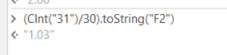# How to divide a number and get results with decimals

my having a number.tostring and i need to divide by 30 (number/30)
but i m not getting the results with decimals i m getting it round-off
Ex - 31/30=1
how can i get the result with decimals to write it to a cell

Results = 31/30=1.03

Thank you

You can try the below…

``````decimal.divide(31,30).tostring("#.##")
``````

OutputHope this helps…

1 Like

find some basics:and give a try on:2 Likes

This topic was automatically closed 3 days after the last reply. New replies are no longer allowed.